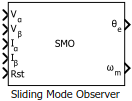# Sliding Mode Observer

Compute electrical position and mechanical speed of a surface-mount PMSM

• Library:
• Motor Control Blockset / Sensorless Estimators

•## Description

The Sliding Mode Observer block computes the electrical position and mechanical speed of a Surface Mount PMSM by using the voltage and current values along the α- and β-axes of the stationary αβ reference frame.

### Equations

These equations describe the discrete-time operation of a PMSM:

`${i}_{\alpha \beta \left(k+1\right)}=A{i}_{\alpha \beta \left(k\right)}+B{v}_{\alpha \beta \left(k\right)}-B{e}_{\alpha \beta \left(k\right)}$`
`${e}_{\alpha \beta \left(k+1\right)}={e}_{\alpha \beta \left(k\right)}+{T}_{s}{\omega }_{e\left(k\right)}J{e}_{\alpha \beta \left(k\right)}$`
`$J=\left[\begin{array}{cc}0& -1\\ 1& 0\end{array}\right]$`
`$\Phi =\left[\begin{array}{cc}-\frac{R}{L}& 0\\ 0& -\frac{R}{L}\end{array}\right]$`
`$A={e}^{\Phi {T}_{s}}$`
`$B=\underset{0}{\overset{{T}_{s}}{\int }}{e}^{\Phi \tau }d\tau =\left[\begin{array}{cc}b& 0\\ 0& b\end{array}\right]$`
`$b=\frac{1-{e}^{-R{T}_{s}/L}}{R}$`

These equations describe the discrete-time sliding mode observer operation of a surface mount PMSM:

`${\stackrel{^}{i}}_{\alpha \beta \left(k+1\right)}=A{\stackrel{^}{i}}_{\alpha \beta \left(k\right)}+B{v}_{\alpha \beta \left(k\right)}-B{\stackrel{^}{e}}_{\alpha \beta \left(k\right)}-\eta Sign\left({\stackrel{˜}{i}}_{\alpha \beta \left(k\right)}\right)$`
`${\stackrel{^}{e}}_{\alpha \beta \left(k+1\right)}={\stackrel{^}{e}}_{\alpha \beta \left(k\right)}+{B}^{-1}g\left({\stackrel{˜}{i}}_{\alpha \beta \left(k\right)}-A{\stackrel{˜}{i}}_{\alpha \beta \left(k-1\right)}+\eta Sign\left({\stackrel{˜}{i}}_{\alpha \beta \left(k-1\right)}\right)\right)$`
`${\stackrel{˜}{i}}_{\alpha \beta \left(k\right)}={\stackrel{^}{i}}_{\alpha \beta \left(k\right)}-{i}_{\alpha \beta \left(k\right)}$`
`${\stackrel{˜}{e}}_{\alpha \beta \left(k\right)}={\stackrel{^}{e}}_{\alpha \beta \left(k\right)}-{e}_{\alpha \beta \left(k\right)}$`

If the back EMF observer fulfils the conditions $|{e}_{\alpha \beta \left(k+1\right)}-{e}_{\alpha \beta \left(k\right)}|\le m$ and $g\in \left(0,1\right)$, there exists a k0, such that:

`${\stackrel{˜}{e}}_{\alpha \beta \left(k\right)}<\frac{m}{g}$`

If the sliding mode observer fulfils these conditions:

• $g\in \left(0,1\right)$

• $|{e}_{\alpha \beta \left(k+1\right)}-{e}_{\alpha \beta \left(k\right)}|\le m$

• $\eta >b\frac{m}{g}$

then there exists a k=k0, such that for k≥k0:

`$|{\stackrel{˜}{i}}_{\alpha \beta \left(k\right)}|\le \eta +b\frac{m}{g}$`

where:

• eα and iα are the stator back EMF and current for the α axis

• eβ and iβ are the stator back EMF and current for the β axis

• α and ĩα are the errors in the stator back EMF and current for the α axis

• β and ĩβ are the errors in the stator back EMF and current for the β axis

• vα and vβ are the stator supply voltages

• R is the stator resistance

• L is the stator inductance

• g is the back EMF observer gain

• η is the current observer gain

• ωe is the electrical angular velocity

• Ts is the sampling period

• k is the sample count

### Tuning

Use these steps to tune the block using the Current observer gain (η) and Back-emf observer gain (g) parameters.

• Select a back-emf observer gain (g) value such that $g\in \left(0,1\right)$. Bringing g close to the value `1`, results in less error in the estimated back-emf. However, this makes convergence slow.

• Select a value of m based on the block sample time and maximum slope of the operating back-emf (such that $|{e}_{\alpha \beta \left(k+1\right)}-{e}_{\alpha \beta \left(k\right)}|\le m$).

• Select a current observer gain (η) value based on b, m, and g (such that $\eta >b\frac{m}{g}$).

Note

The block functions correctly when you tune the sliding mode observer gains.

When using open-loop control to run a motor, compute the rotor position using both sliding mode observer and an actual sensor hardware and compare the computed position values. If the difference is acceptable, the block functions correctly. Otherwise, manually tune the sliding mode observer gains to ensure that the block functions accurately.

The transition from open-loop control to closed-loop control may fail due to noise in the currents and voltages. To make a successful transition, try reducing the value of the Filter cut-off frequency (Hz) parameter.

## Ports

### Input

expand all

Voltage component along the α-axis of the stationary αβ reference frame.

Data Types: `single` | `double` | `fixed point`

Voltage component along the β-axis of the stationary αβ reference frame.

Data Types: `single` | `double` | `fixed point`

Current component along the α-axis of the stationary αβ reference frame.

Data Types: `single` | `double` | `fixed point`

Current component along the β-axis of the stationary αβ reference frame.

Data Types: `single` | `double` | `fixed point`

The pulse (true value) that resets and restarts the processing of the block algorithm.

Data Types: `single` | `double` | `fixed point`

### Output

expand all

The estimated electrical position of the rotor.

Data Types: `single` | `double` | `fixed point`

The estimated mechanical speed of the rotor.

Data Types: `single` | `double` | `fixed point`

## Parameters

expand all

Unit of the input voltage and current components along the α-axis and β-axis of the stationary αβ reference frame.

The fixed time interval (in seconds) between every two consecutive instances of block execution.

### Motor parameters

Stator phase winding resistance (in ohm).

Stator phase winding inductance (in Henry).

Maximum speed (in RPM) that the block can support. For a speed beyond this value, the block generates incorrect outputs.

Number of pole pairs available in the motor.

The maximum phase voltage applied to the PMSM. For details, see Per-Unit System.

The maximum measurable current supplied to the PMSM. For details, see Per-Unit System.

Note

The Sliding Mode Observer block might occasionally display the warning message `'Wrap on overflow detected`.`'`

### Observer Parameters

The gain that ensures the convergence of the back-emf observer.

The gain that ensures the convergence of the current observer.

The cut-off frequency of the internal low-pass IIR filter. The cut-off frequency value must be greater than or equal to the maximum electrical frequency.

Click to calculate approximate observer gains and the filter coefficient and update these fields. For this calculation, we set g to `0.9`, computed m at twice the rated speed, and set η to $1.1\left(b\frac{m}{g}\right)$.

### Datatypes

Unit of the position output.

Data type of the position output.

Unit of the speed output.

Data type of the speed output.

 T. Bernardes, V. F. Montagner, H. A. Gründling, and H. Pinheiro, "Discrete-Time Sliding Mode Observer for Sensorless Vector Control of Permanent Magnet Synchronous Machine," in IEEE Transactions on Industrial Electronics, vol. 61, no. 4, pp. 1679-1691, 2014

 B. Bose, Modern Power Electronics and AC Drives. Prentice Hall, 2001. ISBN-0-13-016743-6.

 J. Liu and X. Wan, "Advanced Sliding Mode Control for Mechanical Systems". Springer-Verlag Berlin Heidelberg, 2011.